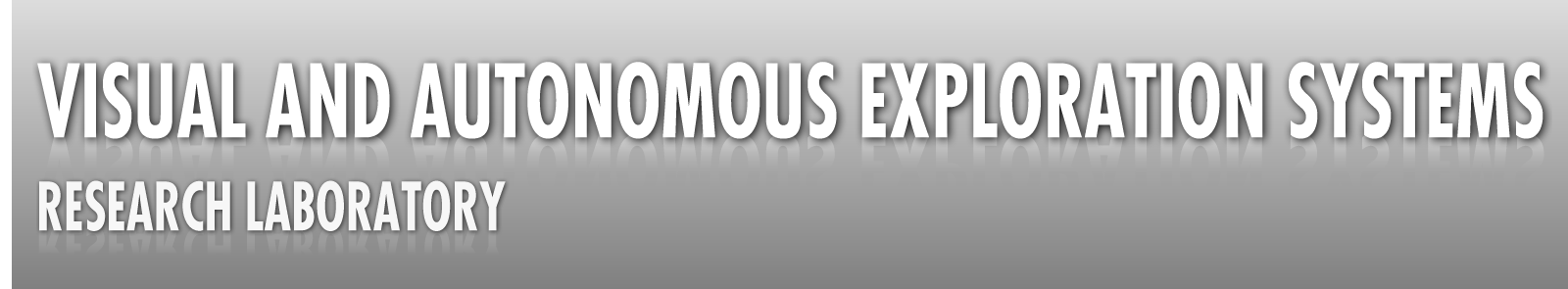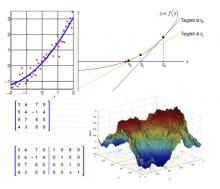# CoursesECE 411/511: Numeric Modeling of Physics & Biological Systems
Offered: Fall
Technical Elective

This course combines themes from mechanics, electromagnetics, thermal physics, and neural networks with an introduction to numerical methods as well as the use of MATLAB. Students will become familiar with the underlying theory for a variety of systems in physics and biology (e.g., harmonic, anharmonic and coupled oscillators; electric fields of electric lenses; geo-thermal power station; and artificial neural networks), derive the necessary mathematical equations describing these systems, learn the necessary numerical methods to solve the underlying equations, and implement the system equations and numerical methods in MATLAB to simulate these systems. As a result, students will be prepared to formulate problems or model systems in physics, biology, and related disciplines, and to solve them numerically or in simulation.ECE 330: Computational Techniques
Offered: Spring
Technical Elective

This course provides students with the fundamentals of computational techniques for solving numerical problems. In particular students will become familiar with techniques for numerical differentiation, numerical integration, solving differential equations (e.g., Runge-Kutta method), solving systems of linear equations (e.g., Gauss-Jordan elimination), discretization of differential operators, aspects of numerical linear algebra (e.g., matrix inversion), root finding (e.g., Newton-Raphson method), and numerical optimization (least squares method, linear programming, and stochastic optimization techniques such as simulated annealing and genetic algorithms). In addition students will be provided with a basic working knowledge of the Matlab environment: They will learn how to create, edit, compile, and run programs in Matlab. Moreover, students will be provided with a basic working knowledge of Gnuplot: They will become familiar with 2D and 3D plotting techniques. Furthermore, students will be introduced to Numerical Recipes and the GNU Scientific Library.